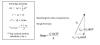﻿ Untitled 1 Chapter 3 Test Information
• Test format:
• 4 short answer
• 8 multiple choice, including basic vector problems (adding vectors to get resultant magnitude and direction, resolving vector into components)
• 2 River problems (2-3 parts each) -- Constant velocity in 2 dimensions
• 3 Projectile Problems (2-4 parts each) -- Kinematics in 2-D with acceleration due to gravity.
• Sources for Similar Problems/Questions:
• Some Important Concepts:
• Memorize -- 1m/s ≈ 2.24mph.
• Add vectors head-to-tail
• Determine the magnitude and direction of a vector, given its components (e.g. "degrees above +X" or "degrees north of west")
• Find the missing vector using the concept of head-to tail addition
• "River problems"
• Identifying the resultant and component vectors
• Solve for one of these: current or wind speed and/or direction, object speed and heading relative to water or air, actual object velocity relative to the earth
• Motion in terms of x and y...
• Remember the independence of x and y components of projectile behavior
• Resolving a vector into x and y components
• Adding x and y vectors to get a magnitude and direction of a resultant
• SohCahToa
• Finding angles with inverse functions.
• Narrate what happens to vx and vy at all points during the flight of a projectile and explain why.
• Determine the following for a projectile:
•  x or y displacement  (given initial direction, initial velocity, and initial position)
• time aloft
• x or y velocity
• speed and direction
• Provided formulas: